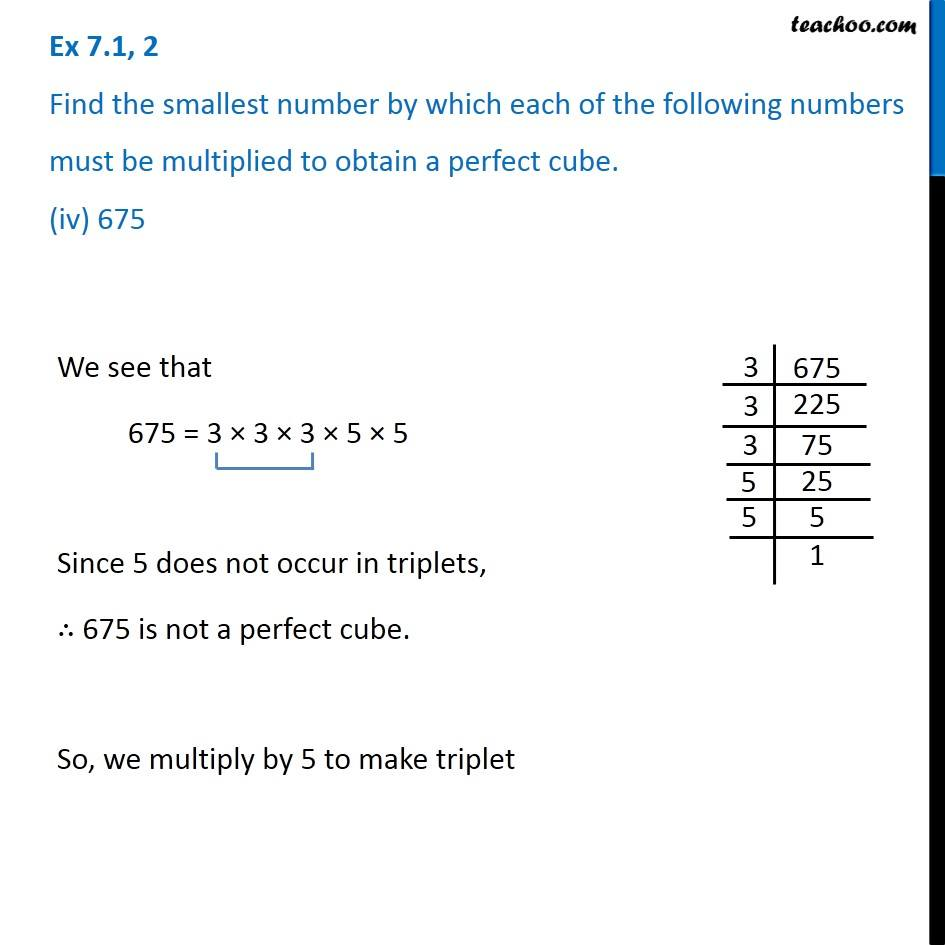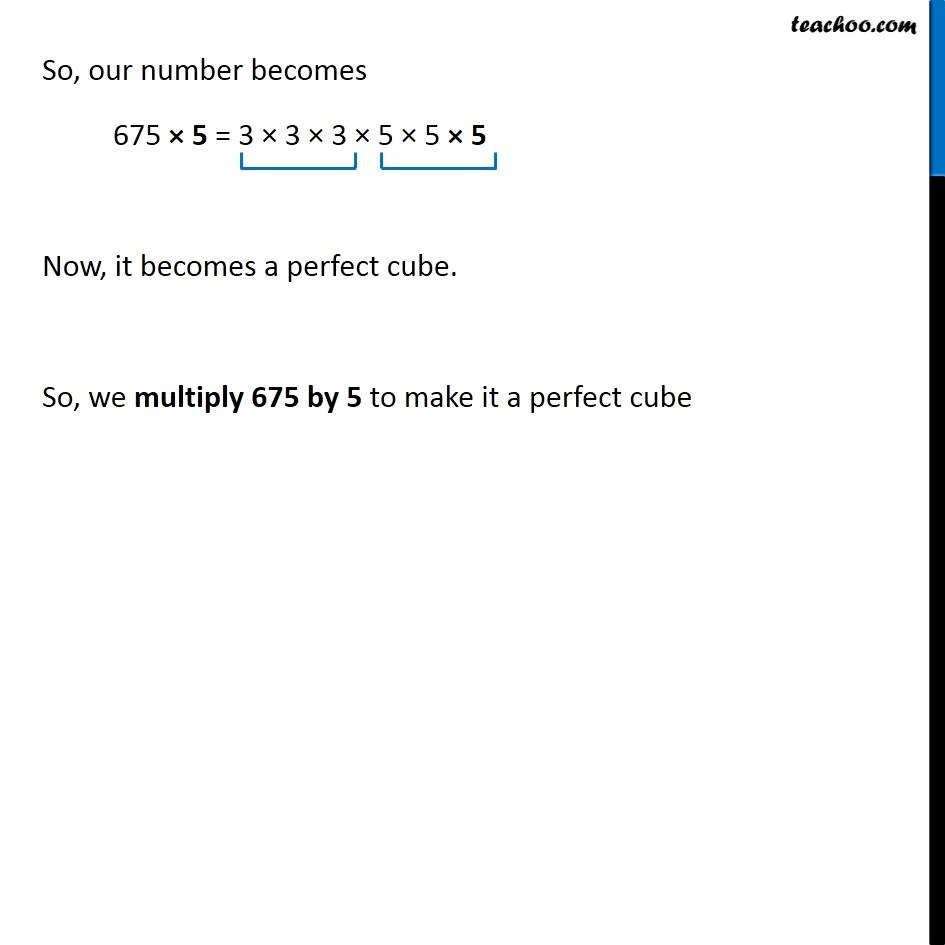Ex 7.1

Chapter 7 Class 8 Cubes and Cube Roots
Serial order wiseGet live Maths 1-on-1 Classs - Class 6 to 12

### Transcript

Ex 7.1, 2 Find the smallest number by which each of the following numbers must be multiplied to obtain a perfect cube. (iv) 675 We see that 675 = 3 × 3 × 3 × 5 × 5 Since 5 does not occur in triplets, ∴ 675 is not a perfect cube. So, we multiply by 5 to make triplet So, our number becomes 675 × 5 = 3 × 3 × 3 × 5 × 5 × 5 Now, it becomes a perfect cube. So, we multiply 675 by 5 to make it a perfect cube